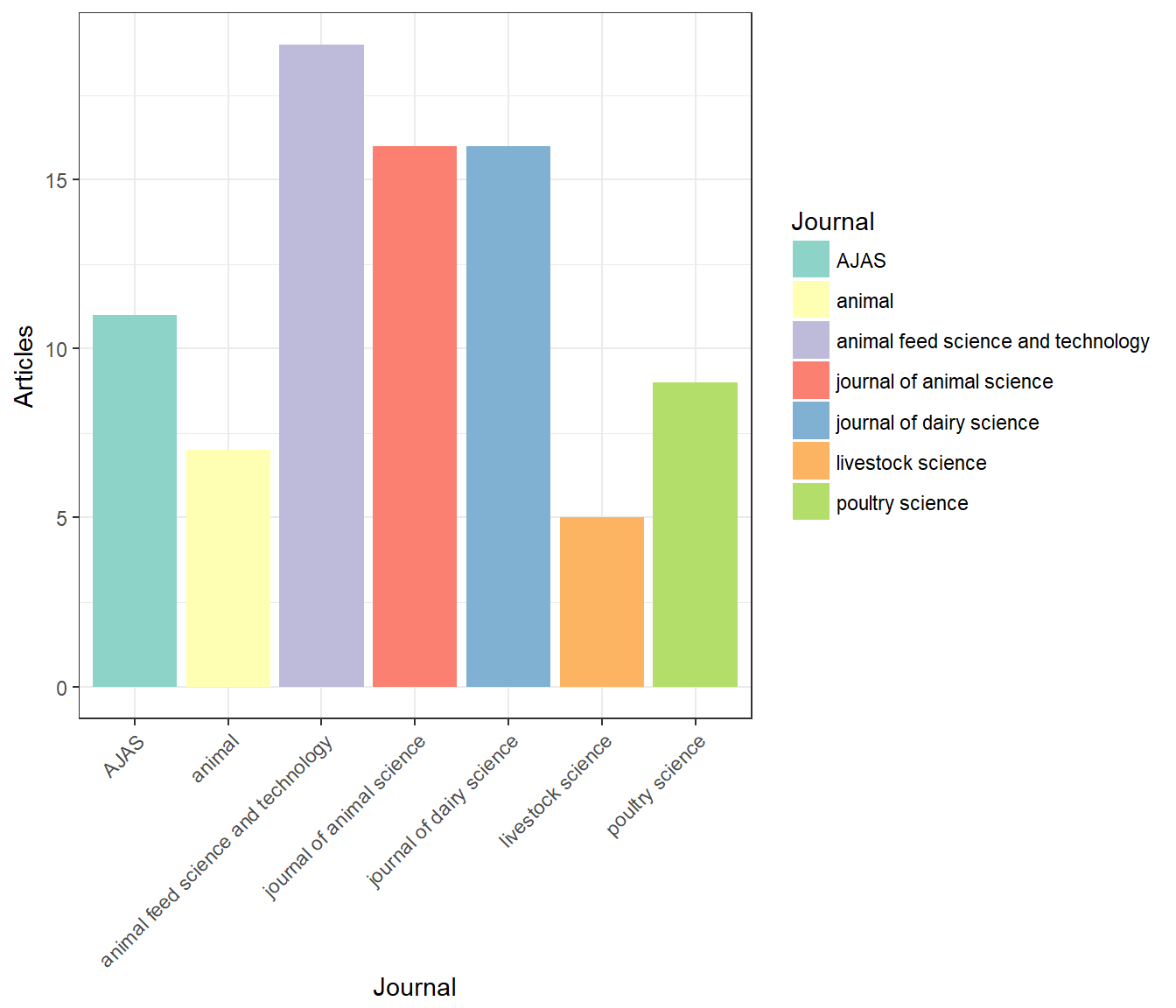Animal nutrition journal report: February 2018

A report is published for a personal interesting of the recent animal nutrition. The papers about “animal nutrition” which published at some SCI(E) journals were selected and analyzed. HTML scraping, data visualization, English-Korean translation, and report generation are automate process except sorting of the papers. Codes are available at https://github.com/YoungjunNa/journal_scraping

# 1. List of journals

``````journal_list <- readxl::read_excel("journal_result_2018_2.xlsx")
table <- table(journal_list\$journal) %>% data.frame()
colnames(table) <- c("Journal","Articles")
kable(table)``````
Journal Articles
AJAS 11
animal 7
animal feed science and technology 19
journal of animal science 16
journal of dairy science 16
livestock science 5
poultry science 9
``ggplot(table, aes(x=Journal, y=Articles, fill=Journal)) + geom_col() + theme(axis.text.x=element_text(angle=45, hjust=1)) + scale_fill_brewer(palette="Set3")``# 2. List of papers

## 2.1. Review

``````results <- readxl::read_excel("journal_result_2018_2.xlsx")
results <- results[,c(7,9,8,5,4,1,2,3,6)]
filter(results, type=="review")``````

## 2.2. Monogastric

``filter(results, type=="original article" & class=="monogastric")``

## 2.3. Ruminant

``filter(results, type=="original article" & class=="ruminant")``

# 3. Wordcloud analysis

## 3.1. Monogastric

``````wc <- readxl::read_excel("journal_result_2018_2.xlsx")
#wc <- filter(wc, class == "ruminant")
wc <- filter(wc, class == "monogastric")
#wc <- filter(wc, str_detect(wc\$subject,"supplement")==TRUE)

#WORDCLOUD
wc <- Corpus(VectorSource(wc\$subject))

wc_data<-tm_map(wc,stripWhitespace)
wc_data<-tm_map(wc_data, removeWords, c("and"))

wc_data<-tm_map(wc_data, tolower)
wc_data<-tm_map(wc_data,removeNumbers)
wc_data<-tm_map(wc_data, removePunctuation)
wc_data<-tm_map(wc_data, removeWords, stopwords("english"))
wc_data<-tm_map(wc_data, removeWords, c("affect","effect","effects","and","the","our","that","for","are","also","more","has","must","have","should","this","with"))

tdm_wc<-TermDocumentMatrix(wc_data) #Creates a TDM
TDM1<-as.matrix(tdm_wc) #Convert this into a matrix format
v = sort(rowSums(TDM1), decreasing = TRUE) #Gives you the frequencies for every word

wordcloud(wc_data, max.words = Inf, min.freq = 1, random.order = FALSE, rot.per = 0.1, colors = brewer.pal(8, "Dark2")) ``````